# Vibrations of the nonlinear system in which stationary harmonic excited multivalued regimes in the vicinities of resonances do not exist

## K. Ragulskis1, L. Ragulskis2

1Kaunas University of Technology, K. Donelaičio Str. 73, LT-44249, Kaunas, Lithuania

2Vytautas Magnus University, Vileikos Str. 8, LT-44404, Kaunas, Lithuania

1Corresponding author

Mathematical Models in Engineering, Vol. 5, Issue 3, 2019, p. 97-104. https://doi.org/10.21595/mme.2019.20942
Received 15 July 2019; received in revised form 11 August 2019; accepted 20 August 2019; published 30 September 2019

Copyright © 2019 K. Ragulskis, et al. This is an open access article distributed under the Creative Commons Attribution License, which permits unrestricted use, distribution, and reproduction in any medium, provided the original work is properly cited.
Views 35
CrossRef Citations 0
Abstract.

A nonlinear dynamical system is investigated which consists from a mass between two linear elastic connecting elements with different coefficients of stiffness. Laws of vibrations and characteristics of eigenvibrations of the system as well as of self-decaying vibrations of the system with damping and of the system with harmonic excitation are determined. Dynamical qualities of the system are revealed. It is shown that the system has infinite number of eigenfrequencies and that in the resonance zones multivalued stable and unstable motions do not exist in the system.

Keywords: nonlinear system, coefficients of stiffness, amplitude-frequency characteristics, dynamical qualities.

#### 1. Introduction

Basic concepts of investigation of vibrating systems are presented in . Stabilisation of periodic nonlinear systems is investigated in . Frequency analysis of a typical system is performed in . Pendulum mechanism is investigated in . Nonlinear vibrations of a piecewise linear model are analysed in . Multiple resonant zones are investigated in . Sommerfeld effect is analysed in . Isolated resonances are investigated in . Dynamics of vibromotors is analysed in .

This paper is dedicated for the investigation of a nonlinear system which does not possess multivalued regimes. Moreover, amplitude-frequency characteristics of such kind of system are linear. Such types of systems are important from the point of view of different engineering applications where stationary regimes do posses the stability in respect to different law of motions and energy regimes. The governing system of equations describing the investigated class of systems reads:

(1)
(2)

It is assumed that:

(3)

#### 2. The conservative system

In this case in the Eqs. (1), (2) it is assumed that:

(4)

According to the Eqs. (1), (4) when the initial conditions of motion are:

(5)

it is obtained in the interval $t\in \left[0,{\stackrel{-}{T}}_{1}\right]:$

(6)
$\begin{array}{l}x=-\frac{{\stackrel{˙}{x}}^{+}}{p}\mathrm{s}\mathrm{i}\mathrm{n}{p}_{1}t,\\ \stackrel{˙}{x}=-{\stackrel{˙}{x}}^{+}\mathrm{c}\mathrm{o}\mathrm{s}{p}_{1}t.\end{array}$

From where it is obtained:

(7)
${\stackrel{-}{T}}_{1}=\frac{\pi }{{p}_{1}}.$

Motion in the interval:

(8)
$t\in \left[{\stackrel{-}{T}}_{1},{\stackrel{-}{T}}_{1}+{T}_{2}\right],$

takes place continuously, that is at $t={\stackrel{-}{T}}_{1}:x=0$, $\stackrel{˙}{x}={\stackrel{˙}{x}}^{-}.$ According to the Eqs. (2), (4):

(9)
$\begin{array}{l}x=\frac{{\stackrel{˙}{x}}^{-}}{{p}_{2}}\mathrm{s}\mathrm{i}\mathrm{n}{p}_{2}\left(t-{\stackrel{-}{T}}_{1}\right),\\ \stackrel{˙}{x}={\stackrel{˙}{x}}^{-}\mathrm{c}\mathrm{o}\mathrm{s}{p}_{2}\left(t-{\stackrel{-}{T}}_{1}\right).\end{array}$

From the Eqs. (8), (9):

(10)
${\stackrel{-}{T}}_{2}=\frac{\pi }{{p}_{2}},$

and:

(11)
$\stackrel{-}{T}=\pi \frac{{p}_{1}+{p}_{2}}{{p}_{1}{p}_{2}}=\frac{2\pi }{\stackrel{-}{\omega }},$

where the frequency of eigenvibrations $\stackrel{-}{\omega }:$

(12)
$\stackrel{-}{\omega }=2\frac{{p}_{1}{p}_{2}}{{p}_{1}+{p}_{2}}.$

The whole solution by performing general notation $x$ is obtained:

(13)
(14)
(15)

Further $x$, $\stackrel{˙}{x}$, $\stackrel{¨}{x}$ by taking into account the Eqs. (13)-(15) expansion into the Fourier series with respect to $\stackrel{-}{\omega }$ is performed:

(16)

and the following relationships are obtained:

(17)

where ${x}_{a}$ is the maximum deviation from the medium value of the whole process, ${x}_{na}$ are the amplitudes of the respective harmonics. In the same way the expansions of $\stackrel{˙}{x}$ and $\stackrel{¨}{x}$ are performed.

According to the Eqs. (13)-(17) by assuming:

(18)

and the initial conditions of motion at $t=0:$

(19)

graphical relationships are obtained $x=x\left(t\right)\text{,}$$\stackrel{˙}{x}=\stackrel{˙}{x}\left(t\right)\text{,}$$\stackrel{¨}{x}=\stackrel{¨}{x}\left(t\right)\text{,}$$\stackrel{˙}{x}\stackrel{¨}{x}=\stackrel{˙}{x}\stackrel{¨}{x}\left(t\right)\text{,}$$\stackrel{˙}{x}=\stackrel{˙}{x}\left(x\right)\text{,}$$\stackrel{¨}{x}=\stackrel{¨}{x}\left(\stackrel{˙}{x}\right)$, $\stackrel{˙}{x}\stackrel{¨}{x}=\stackrel{˙}{x}\stackrel{¨}{x}\left(x\right)$ (see Fig. 1).

Fig. 1. Dynamics of the system for the initial conditions of motion $t=\text{0}$, $x\left(0\right)=\text{0}$, –1 (thin line), $t=\text{0}$, $x\left(0\right)=\text{0}$, $\stackrel{˙}{x}\left(0\right)=$ –2/3 (line of medium thickness) and $t=\text{0}$, $x\left(0\right)=\text{0}$, $\stackrel{˙}{x}\left(0\right)=$ –1/3 (thick line)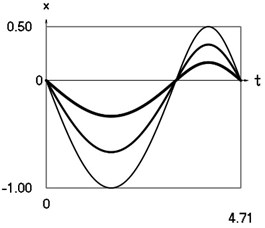a) Displacement as function of time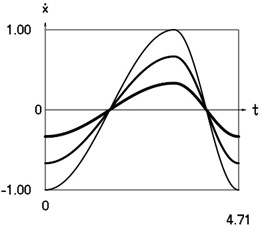b) Velocity as function of time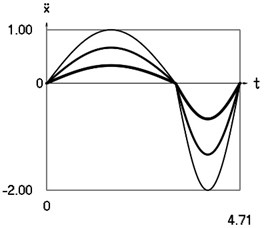c) Acceleration as function of time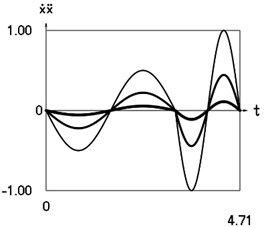d) Velocity multiplied by acceleration as function of time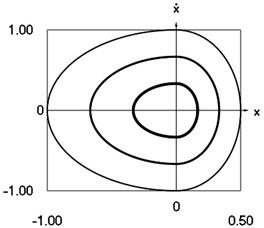e) Phase trajectory: velocity as function of displacement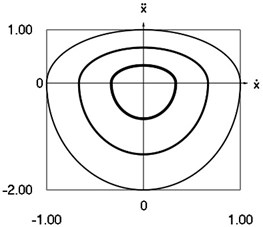f) Phase trajectory: acceleration as function of velocity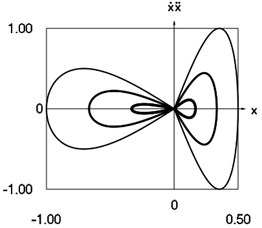g) Phase trajectory: velocity multiplied by acceleration as function of displacement

According to the Eq. (12) eigenfrequency of the system:

(20)
$\stackrel{-}{\omega }=2\frac{1\cdot 2}{1+2}=\frac{4}{3}.$

In the expansions into the Fourier series the first four members $n=0,1,2,3$ are taken into account.

By taking into account the Eqs. (11), (12) Table 1 is obtained.

According to the Eqs. (13)-(17) by taking into account Eqs. (18)-(20) graphical relationships are obtained (see Fig. 2).

Table 1. Analysis of harmonics

 $n$ 0 1 2 3 … $n$ $n\stackrel{-}{\omega }$ 0 $\frac{4}{3}$ $2\cdot \frac{4}{3}$ $3\cdot \frac{4}{3}$ … $n\cdot \frac{4}{3}$ ${\stackrel{-}{T}}_{n}=\frac{2\pi }{n\stackrel{-}{\omega }}$ $\infty$ $\frac{3}{2}\pi$ $\frac{3}{2\cdot 2}\pi$ $\frac{3}{3\cdot 2}\pi$ … $\frac{3}{n\cdot 2}\pi$

Fig. 2. Amplitude frequency characteristics (constant part and first three harmonics)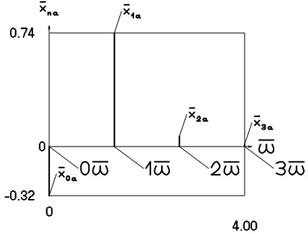a) Displacement frequency characteristic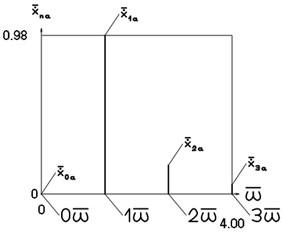b) Velocity frequency characteristic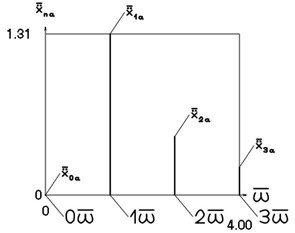c) Acceleration frequency characteristic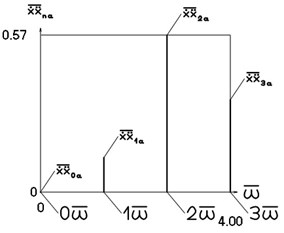d) Velocity multiplied by acceleration frequency characteristic

#### 3. Forced vibrations

According to the Eqs. (1), (2) by assuming:

(21)
${p}_{1}=1,{p}_{2}=2,{h}_{1}=0.1,{h}_{2}=0.2,f\equiv \mathrm{v}\mathrm{a}\mathrm{r},$

and at:

(22)
$\omega =\frac{3}{4}\stackrel{-}{\omega },\stackrel{-}{\omega },\frac{4}{3}\stackrel{-}{\omega },\frac{5}{3}\stackrel{-}{\omega },2\stackrel{-}{\omega },\frac{5}{2}\stackrel{-}{\omega },$

steady state solutions are found and their spectral analysis $\omega$, $2\omega$, $3\omega$, $4\omega$, ... is performed.

Steady state solutions are presented in Fig. 3, Fig. 4, Fig. 5, Fig. 6, Fig. 7, Fig. 8.

Fig. 3. Steady state motions of the system for the first value of frequency of excitation and $f=$ –1 (thin line), $f=$ –2/3 (line of medium thickness), –1/3 (thick line)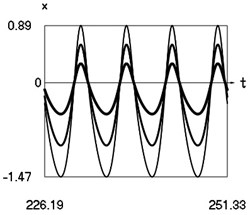a) Displacement as function of time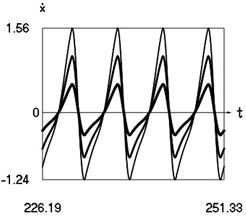b) Velocity as function of time

Fig. 4. Steady state motions of the system for the second value of frequency of excitation and $f=$ –1 (thin line), $f=$ –2/3 (line of medium thickness), –1/3 (thick line)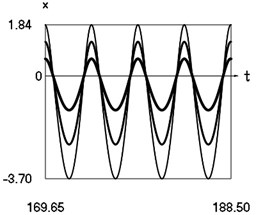a) Displacement as function of time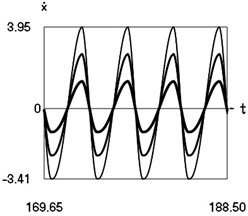b) Velocity as function of time

Fig. 5. Steady state motions of the system for the third value of frequency of excitation and $f=$ –1 (thin line), $f=$ –2/3 (line of medium thickness), –1/3 (thick line)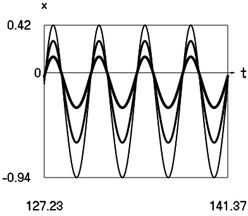a) Displacement as function of time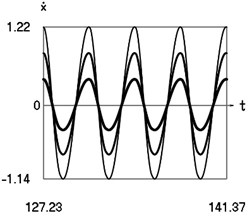b) Velocity as function of time

Fig. 6. Steady state motions of the system for the fourth value of frequency of excitation and $f=$ –1 (thin line), $f=$ –2/3 (line of medium thickness), –1/3 (thick line)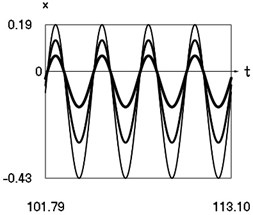a) Displacement as function of time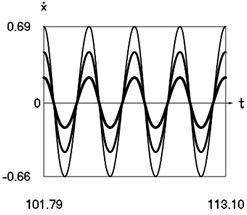b) Velocity as function of time

Fig. 7. Steady state motions of the system for the fifth value of frequency of excitation and $f=$ –1 (thin line), $f=$ –2/3 (line of medium thickness), –1/3 (thick line)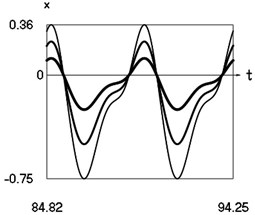a) Displacement as function of time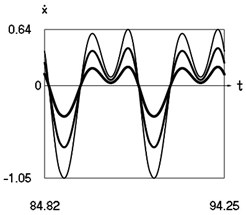b) Velocity as function of time

Fig. 8. Steady state motions of the system for the sixth value of frequency of excitation and $f=$ –1 (thin line), $f=$ –2/3 (line of medium thickness), –1/3 (thick line)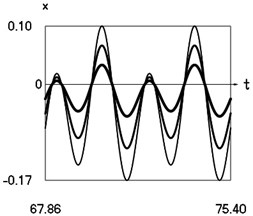a) Displacement as function of time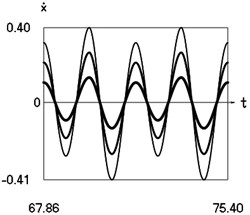b) Velocity as function of time

Amplitude frequency characteristics are presented in Fig. 9, Fig. 10, Fig. 11, Fig. 12, Fig. 13, Fig. 14.

Fig. 9. Amplitude frequency characteristics (constant part and first three harmonics) for the first value of frequency of excitation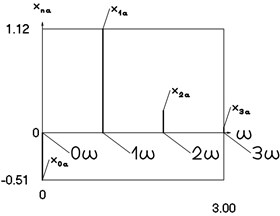a) Displacement frequency characteristic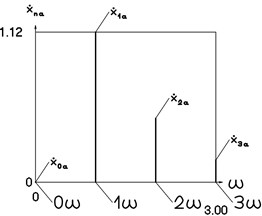b) Velocity frequency characteristic

Fig. 10. Amplitude frequency characteristics (constant part and first three harmonics) for the second value of frequency of excitation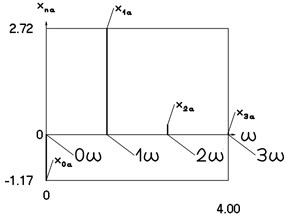a) Displacement frequency characteristic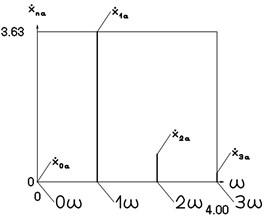b) Velocity frequency characteristic

Fig. 11. Amplitude frequency characteristics (constant part and first three harmonics) for the third value of frequency of excitation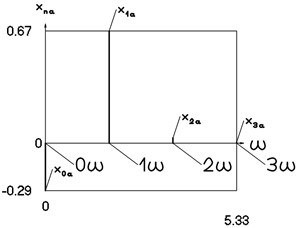a) Displacement frequency characteristic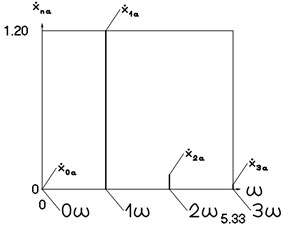b) Velocity frequency characteristic

Fig. 12. Amplitude frequency characteristics (constant part and first three harmonics) for the fourth value of frequency of excitation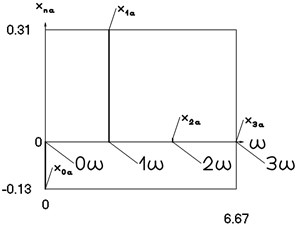a) Displacement frequency characteristic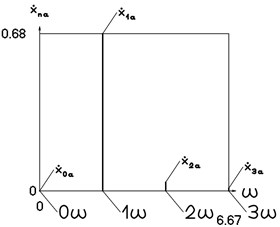b) Velocity frequency characteristic

Fig. 13. Amplitude frequency characteristics (constant part and first three harmonics) for the fifth value of frequency of excitation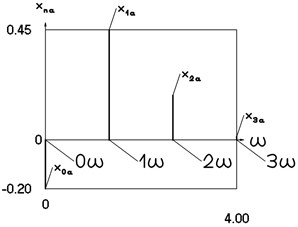a) Displacement frequency characteristic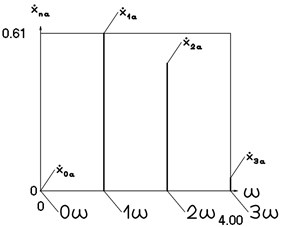b) Velocity frequency characteristic

Fig. 14. Amplitude frequency characteristics (constant part and first three harmonics) for the sixth value of frequency of excitation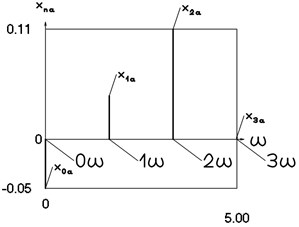a) Displacement frequency characteristic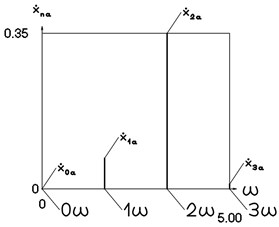b) Velocity frequency characteristic

#### 4. Conclusions

On the basis of the presented results the qualities of dynamic behavior of the nonlinear vibrating system which consists from a mass between two linear elastic connecting members with different coefficients of stiffness can be used in different engineering applications requiring the stability in a wide range of parameters.

The motions of the system and amplitude frequency characteristics are determined by analytical – numerical method relationships. It is determined that for the case of the conservative system eigenfrequencies do not depend on the value of the amplitude of excitation. It is shown that for the case of forced harmonic excitation stable and unstable multivalued regimes do not exist in the system. Also, separate classes of nonlinear dynamical systems are investigated which have qualities of similar type.

The presented results enable to perform the design of nonlinear vibrating systems of this type. This enables to create new systems which can be noted by high stability properties, because in the vicinities of resonances they do not have multivalued solutions.

1. Bolotin V. V. Vibrations in Engineering. Handbook, Vol. 1, Mashinostroienie, Moscow, 1978, (in Russian). [Search CrossRef]
2. Zaitsev V. A. Global asymptotic stabilization of periodic nonlinear systems with stable free dynamics. Systems and Control Letters, Vol. 91, 2016, p. 7-13. [Publisher]
3. Salahshoor E., Ebrahimi S., Zhang Y. Frequency analysis of a typical planar flexible multibody system with joint clearances. Mechanism and Machine Theory, Vol. 126, 2018, p. 429-456. [Publisher]
4. Starossek U. Forced response of low-frequency pendulum mechanism. Mechanism and Machine Theory, Vol. 99, 2016, p. 207-216. [Publisher]
5. Wang S., Hua L., Yang C., Zhang Y., Tan X. Nonlinear vibrations of a piecewise-linear quarter-car truck model by incremental harmonic balance method. Nonlinear Dynamics, Vol. 92, 2018, p. 1719-1732. [Publisher]
6. Alevras P., Theodossiades S., Rahnejat H. On the dynamics of a nonlinear energy harvester with multiple resonant zones. Nonlinear Dynamics, Vol. 92, 2018, p. 1271-1286. [Publisher]
7. Sinha A., Bharti S. K., Samantaray A. K., Chakraborty G., Bhattacharyya R. Sommerfeld effect in an oscillator with a reciprocating mass. Nonlinear Dynamics, Vol. 93, 2018, p. 1719-1739. [Publisher]
8. Habib G., Cirillo G. I., Kerschen G. Isolated resonances and nonlinear damping. Nonlinear Dynamics, Vol. 93, 2018, p. 979-994. [Publisher]
9. Ragulskis K., Bansevičius R., Barauskas R., Kulvietis G. Vibromotors for Precision Microrobots. Hemisphere, New York, 1987. [Search CrossRef]